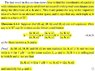# Scalar triple product and abstract vector space

• PcumP_Ravenclaw

#### PcumP_Ravenclaw

Dear all,
Can anyone please explain how the linear combination of non-coplanar and non-orthogonal coordinate axes representing a point x as shown below is derived. Please use the reference text attached in this post to explain to me as i will find it a bit relevant. I want to know how it is derived using the scalar triple product and to get an understanding of what a scalar triple product does to three vectors apart from it calculating the volume of the parrellelepiped formed from the three vectors.

##
x = \dfrac{[x,b,c]}{[a,b,c]} a + \dfrac{[a,x,c]}{[a,b,c]} b + \dfrac{[a,b,x]}{[a,b,c]} c
##

I know that you can just add up the the magnitudes in the directions of the axes a,b and c to locate a point x. but how did the magnitudes in the direction a,b and c come to be

## \dfrac{[x,b,c]}{[a,b,c]} , \dfrac{[a,x,c]}{[a,b,c]} and \dfrac{[a,b,x]}{[a,b,c]} ##

Danke...

#### Attachments

•Untitled (2).jpg
51.5 KB · Views: 623
Dear all,
Can anyone please explain how the linear combination of non-coplanar and non-orthogonal coordinate axes representing a point x as shown below is derived. Please use the reference text attached in this post to explain to me as i will find it a bit relevant. I want to know how it is derived using the scalar triple product and to get an understanding of what a scalar triple product does to three vectors apart from it calculating the volume of the parrellelepiped formed from the three vectors.

##
x = \dfrac{[x,b,c]}{[a,b,c]} a + \dfrac{[a,x,c]}{[a,b,c]} b + \dfrac{[a,b,x]}{[a,b,c]} c
##

I know that you can just add up the the magnitudes in the directions of the axes a,b and c to locate a point x. but how did the magnitudes in the direction a,b and c come to be

## \dfrac{[x,b,c]}{[a,b,c]} , \dfrac{[a,x,c]}{[a,b,c]} and \dfrac{[a,b,x]}{[a,b,c]} ##

Danke...
There is some context missing here. What does the notation [0, a] mean? What does the notation [a, b, c] mean?

Also, in the text you provided, it says "not necessarily orthogonal," which is different from non-orthogonal as you wrote.

[0,a] stands for line segment formed from 0 to point a. It is just vector a. [a,b,c] is the standard notation for scalar triple product. ## [a,b,c] = a \cdot (b \times c) ##

The assumption is that ##\vec{x}## can be written as a linear combination of ##\vec{a}, \vec{b}, \text{and } \vec{c}##; that is, ##\vec{x} = \lambda \vec{a} + \mu \vec{b} + \nu \vec{c}##. ([0, a] seems like clumsy notation for ##\vec{a}##, IMO.)

I would start by using the determinant definition of the scalar triple product on this:
$$\frac{[x, b, c]}{[a, b, c]}$$
and using the assumption I listed above.

•PcumP_Ravenclaw
Hey Mark44,

I just finished reading section 4.6 about orientation and determinants in the same book, Basically, the scalar triple product ## [x, b, c] ## is the (3 x 3) determinant of three vectors. It can be positive or negative denoting positive or negative orientation in either side of the two vectors. (by the way, when we say two vectors are positively or negatively orientated when taken their determinant we mean that the vector product of the two vectors has a particular direction (in either side of the plane of the two vectors) and a magnitude. What does it mean when the determinant of 3 vectors which gives a scalar value which is positive or negative based on scalar triple product definition? ). Determinant is the sum of product of the 3 elements from 3 different vectors including the sign of the permutation.

Can you form the connection between the vector cross product of 2 vectors and a (2 x 2) determinant and the scalar triple product and a (3 x 3) determinant?

Danke...